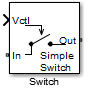# Switch

## Library

Junctions

•## Description

The switch block models a two terminal switch. The input Simulink® signal, Vctl , controls the transfer of the RF Blockset™ signal from the `In` terminal to the `Out` terminal.

If the control voltage is less than the threshold voltage, the block attenuates the signal using the resistance value specified in Off resistance between the input and output terminals. If the control voltage is greater than the threshold voltage, the block passes the signal using the resistance value specified in On resistance between the input and output terminals.

The voltage–current relationship for the switch, or controlled resistor:

• If Vctl < Vthres:

`${I}_{res}.{R}_{off}={V}_{res}$`
• If Vctl Vthres:

`${I}_{res}.{R}_{on}={V}_{res}$`

Roff is the off resistance and Ron is the on resistance of the switch.

If Ron is less than Roff , and Vctl is greater or equal to Vthres, the switch is on.

If Ron is greater than Roff , and Vctl is greater or equal to Vthres, the switch is off.

## Parameters

On resistance

On resistance value of the switch, specified as a positive scalar. The default value is `10`  ohms.

Off resistance

Off resistance value of the switch, specified as a positive scalar. The default value is `1e6`  ohms.

Threshold voltage

Threshold voltage of the switch. The default value is `0`  volts.